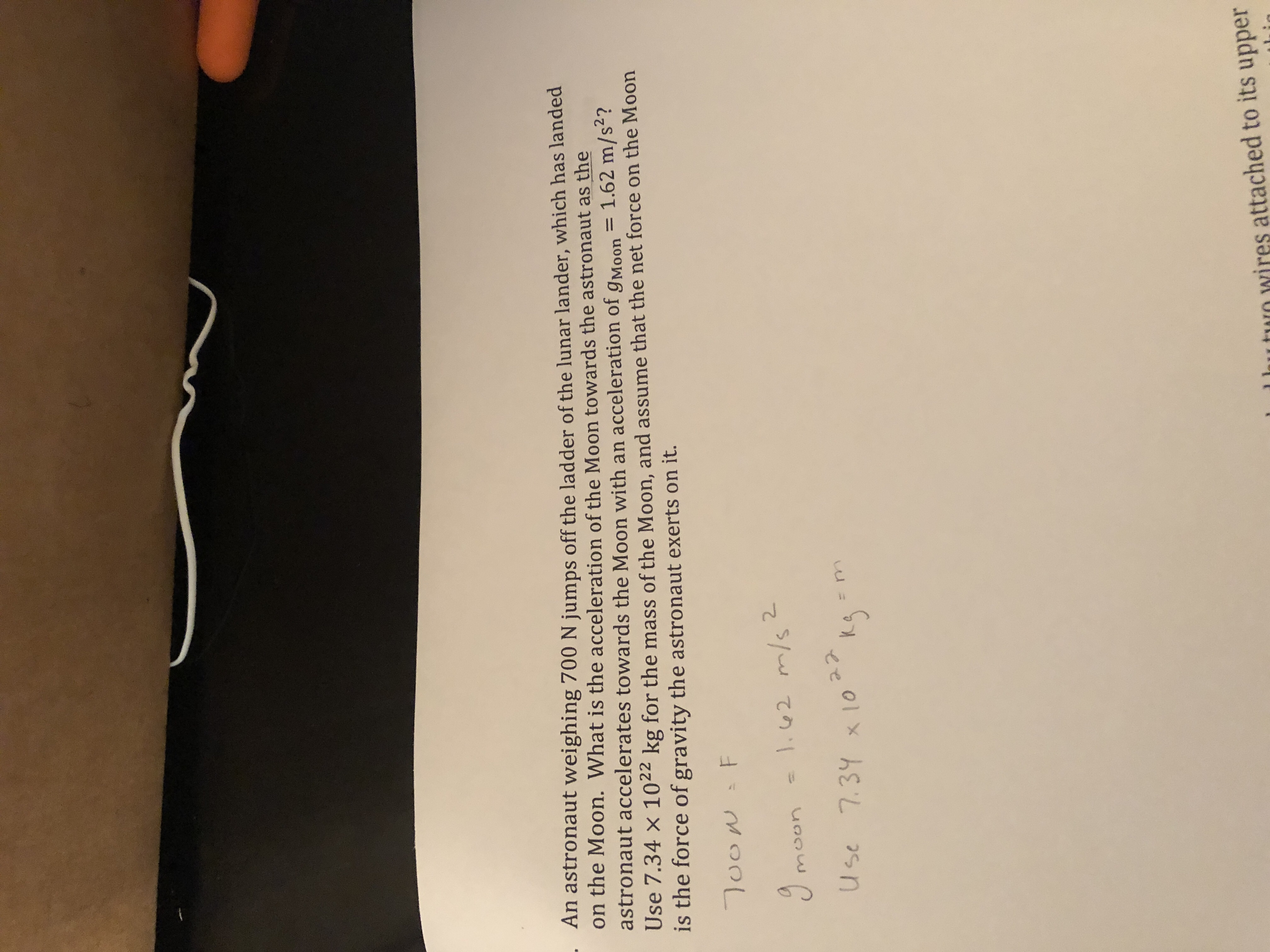An astronaut weighing 700 N jumps off the ladder of the lunar lander, which has landedon the Moon. What is the acceleration of theastronaut accelerates towards the Moon with an acceleration of gMoonUse 7.34 × 1022 kg for the mass of the Moon, and assume that the net force on the Moonis the force of gravity the astronaut exerts on it.Moon towards the astronaut as the2Use 7.31o 5rnl hutun wires attached to its upper

Questionhelp_outlineImage TranscriptioncloseAn astronaut weighing 700 N jumps off the ladder of the lunar lander, which has landed on the Moon. What is the acceleration of the astronaut accelerates towards the Moon with an acceleration of gMoon Use 7.34 × 1022 kg for the mass of the Moon, and assume that the net force on the Moon is the force of gravity the astronaut exerts on it. Moon towards the astronaut as the 2 Use 7.31o 5 rn l hutun wires attached to its upper fullscreen
Step 1

The weight of the astronaut on Earth is 700 N, the acceleration of the astronaut towards the moon is 1.62 m/s2 and the mass of the moon is 7.34×1022 kg.

Step 2

Weight on earth is mass times the gravitational acceleration on earth.

Let W be the weight of the astronaut on earth, mA be the mass of the astronaut and g be the gravitational acceleration on earth.

Write the expression for the weight of the astronaut and rearrange for the mass of the astronaut

Step 3

Substitute 700 N for W and 9.8 m/s2 for in the above eq...

Want to see the full answer?

See Solution

Want to see this answer and more?

Our solutions are written by experts, many with advanced degrees, and available 24/7

See Solution
Tagged in

Physics